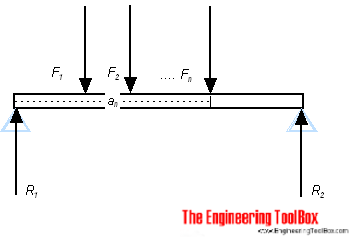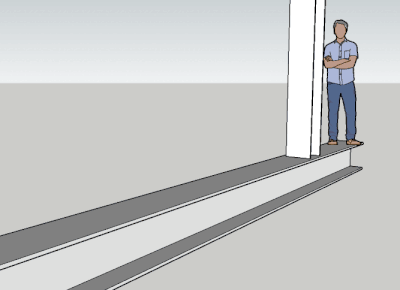Engineering ToolBox - Resources, Tools and Basic Information for Engineering and Design of Technical Applications!

# Beam Loads - Support Force Calculator

## Calculate beam load and supporting forces.### Online Beam Support Force Calculator

The calculator below can be used to calculate the support forces - R1 and R2 - for beams with up to 6 asymmetrically loads.

Length of beam (m, ft)

Force F1 (N, lbf)

Distance from R1 (m, ft)

Force F2 (N, lbf)

Distance from R1 (m, ft)

Force F3 (N, lbf)

Distance from R1 (m, ft)

Force F4 (N, lbf)

Distance from R1 (m, ft)

Force F5 (N, lbf)

Distance from R1 (m, ft)

Force F6 (N, lbf)

Distance from R1 (m, ft)

For a beam in balance loaded with weights (or other load forces) the reactions forces - R - at the supports equals the load forces - F. The force balance can be expressed as

F1 + F2 + .... + Fn = R1 + R2                           (1)

where

F = force from load (N, lbf)

R = force from support (N, lbf)

In addition for a beam in balance the algebraic sum of moments equals zero. The moment balance can be expressed as

F1 af1 + F2 af2 + .... + Fn afn = R ar1 + R ar2                               (2)

where

a = the distance from the force to a common reference - usually the distance to one of the supports (m, ft)

### Example - A beam with two symmetrical loads

A 10 m long beam with two supports is loaded with two equal and symmetrical loads F1 and F2 , each 500 kg. The support forces F3 and F4 can be calculated

(500 kg) (9.81 m/s2) + (500 kg) (9.81 m/s2) = R1 + R2

=>

R1 + R2 = 9810 N

= 9.8 kN

Note! Load due to the weight of a mass - m - is mg Newton's - where g = 9.81 m/s2.

With symmetrical and equal loads the support forces also will be symmetrical and equal. Using

R1 = R2

the equation above can be simplified to

R1 = R2 = (9810 N) / 2

= 4905 N

= 4.9 kN

### Related Mobile Apps from The Engineering ToolBox- free apps for offline use on mobile devices.

### Example - A beam with two not symmetrical loads

A 10 m long beam with two supports is loaded with two loads, 500 kg is located 1 m from the end (R1), and the other load of 1000 kg is located 6 m from the same end. The balance of forces can be expressed as

(500 kg) (9.81 m/s2) + (1000 kg) (9.81 m/s2) = R1 + R2

=>

R1 + R2 = 14715 N

= 14.7 kN

The algebraic sum of moments (2) can be expressed as

(500 kg) (9.81 m/s2) (1 m) + (1000 kg) (9.81 m/s2) (6 m) =?R1 (0 m) + R2 (10 m)

=>

R2 = 6377 (N)

= 6.4 kN

F3 can be calculated as:

R1= (14715 N) - (6377 N)

= 8338 N

= 8.3 kN

### Insert beams to your Sketchup model with the Engineering ToolBox Sketchup Extension## Related Topics

• ### Beams and Columns

Deflection and stress, moment of inertia, section modulus and technical information of beams and columns.
• ### Mechanics

Forces, acceleration, displacement, vectors, motion, momentum, energy of objects and more.
• ### Statics

Loads - forces and torque, beams and columns.

## Related Documents

• ### Aluminum I-Beams

Dimensions and static properties of aluminum I-beams - Imperial units.
• ### American Standard Beams - S Beam

American Standard Beams ASTM A6 - Imperial units.
• ### American Standard Steel C Channels.

Dimensions and static parameters of American Standard Steel C Channels
• ### American Wide Flange Beams

American Wide Flange Beams ASTM A6 in metric units.

• ### British Universal Columns and Beams

Properties of British Universal Steel Columns and Beams.
• ### Cantilever Beams - Moments and Deflections

Maximum reaction forces, deflections and moments - single and uniform loads.
• ### Continuous Beams - Moment and Reaction Support Forces

Moments and reaction support forces with distributed or point loads.
• ### Drawbridge - Force and Moment vs. Elevation

Calculate the acting forces and moments when elevating drawbridges or beams.
• ### Equilibrant Force

The force required to keep a system of forces in equilibrium.
• ### HE-A Steel Beams

Properties of HE-A profiled steel beams.
• ### HE-B Steel Beams

Properties of HE-B profiled steel beams.
• ### HE-M Steel Beams

Properties of HE-M profile steel beams.
• ### Normal Flange I-Beams

Properties of normal flange I profile steel beams.
• ### Square Hollow Structural Sections - HSS

Weight, cross sectional area, moments of inertia - Imperial units
• ### Steel Angles - Equal Legs

Dimensions and static parameters of steel angles with equal legs - imperial units.
• ### Steel Angles - Equal Legs

Dimensions and static parameters of steel angles with equal legs - metric units.
• ### Steel Angles - Unequal Legs

Dimensions and static parameters of steel angles with unequal legs - imperial units.
• ### Steel Angles - Unequal Legs

Dimensions and static parameters of steel angles with unequal legs - metric units.
• ### Steel Pipe Columns - Allowable Loads

Allowable concentric loads for steel pipe columns.
• ### Triangle

Triangle analytical geometry.
• ### Trusses

Common types of trusses.
• ### W-Beams - American Wide Flange Beams

Dimensions of American Wide Flange Beams ASTM A6 (or W-Beams) - Imperial units.
• ### Weight of Beams - Stress and Strain

Stress and deformation of vertical beams due to own weight.

## Engineering ToolBox - SketchUp Extension - Online 3D modeling!

Add standard and customized parametric components - like flange beams, lumbers, piping, stairs and more - to your Sketchup model with the Engineering ToolBox - SketchUp Extension - enabled for use with older versions of the amazing SketchUp Make and the newer "up to date" SketchUp Pro . Add the Engineering ToolBox extension to your SketchUp Make/Pro from the Extension Warehouse !

We don't collect information from our users. More about

## Citation

• The Engineering ToolBox (2009). Beam Loads - Support Force Calculator. [online] Available at: https://www.engineeringtoolbox.com/beams-support-forces-d_1311.html [Accessed Day Month Year].

Modify the access date according your visit.

9.19.12